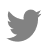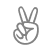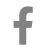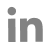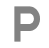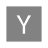# Data Analytics Glossary

Try: Cardinality(SQL), Raw Data, No SQL...

## Confidence Interval

#### What is a Confidence Interval?

A confidence interval, in insights, alludes to the likelihood that a populace boundary will fall between a bunch of values for a specific extent of times. Confidence intervals measure the level of certainty or uncertainty in a sampling technique. They can take quite a few likelihood limits, with the most well-known being a 95% or 99% confidence level.

#### How does a Confidence Interval work?

In doing stats for a set of values statisticians utilized confidence intervals to determine the uncertainty in a sample variable. We understand it's working with an example, suppose a team of researchers is analyzing the heights of the college basketball player. From a random sample taken of the population, researchers found a mean height of 6 feet (72 inches). These 72 inches are an estimation point of the population. Estimation point has limited uses because it is only a random pick, it does not clear certainty or uncertainty. We can assume that the interval is between 70 and 74 inches. From 100 random samples taken from that college, the mean should be between 72 and 76 in 95 percent of those samples.

#### Types and Examples:

1. The confidence interval for the variance

2. The confidence interval for the ratio of two variances

3. The confidence interval for proportions: the binomial proportion confidence interval